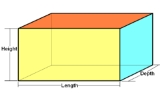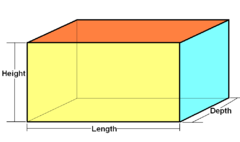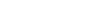xCuboidOverview

In geometry
Geometry
Geometry arose as the field of knowledge dealing with spatial relationships. Geometry was one of the two fields of pre-modern mathematics, the other being the study of numbers ....

, a cuboid is a solid figure bounded by six faces, forming a convex polyhedron. There are two competing (but incompatible) definitions of a cuboid in mathematical literature. In the more general definition of a cuboid, the only additional requirement is that these six faces each be a quadrilateral
Quadrilateral
In Euclidean plane geometry, a quadrilateral is a polygon with four sides and four vertices or corners. Sometimes, the term quadrangle is used, by analogy with triangle, and sometimes tetragon for consistency with pentagon , hexagon and so on...

, and that the undirected graph formed by the vertices and edges of the polyhedron should be isomorphic
Graph isomorphism
In graph theory, an isomorphism of graphs G and H is a bijection between the vertex sets of G and H f \colon V \to V \,\!such that any two vertices u and v of G are adjacent in G if and only if ƒ and ƒ are adjacent in H...

to the graph of a cube
Cube
In geometry, a cube is a three-dimensional solid object bounded by six square faces, facets or sides, with three meeting at each vertex. The cube can also be called a regular hexahedron and is one of the five Platonic solids. It is a special kind of square prism, of rectangular parallelepiped and...

.Unanswered QuestionsWhat is vertical surface area of a cuboid & how the formula is derived ?Encyclopedia
In geometry
Geometry
Geometry arose as the field of knowledge dealing with spatial relationships. Geometry was one of the two fields of pre-modern mathematics, the other being the study of numbers ....

, a cuboid is a solid figure bounded by six faces, forming a convex polyhedron. There are two competing (but incompatible) definitions of a cuboid in mathematical literature. In the more general definition of a cuboid, the only additional requirement is that these six faces each be a quadrilateral
Quadrilateral
In Euclidean plane geometry, a quadrilateral is a polygon with four sides and four vertices or corners. Sometimes, the term quadrangle is used, by analogy with triangle, and sometimes tetragon for consistency with pentagon , hexagon and so on...

, and that the undirected graph formed by the vertices and edges of the polyhedron should be isomorphic
Graph isomorphism
In graph theory, an isomorphism of graphs G and H is a bijection between the vertex sets of G and H f \colon V \to V \,\!such that any two vertices u and v of G are adjacent in G if and only if ƒ and ƒ are adjacent in H...

to the graph of a cube
Cube
In geometry, a cube is a three-dimensional solid object bounded by six square faces, facets or sides, with three meeting at each vertex. The cube can also be called a regular hexahedron and is one of the five Platonic solids. It is a special kind of square prism, of rectangular parallelepiped and...

. Alternatively, the word “cuboid” is sometimes used to refer to a shape of this type in which each of the faces is a rectangle
Rectangle
In Euclidean plane geometry, a rectangle is any quadrilateral with four right angles. The term "oblong" is occasionally used to refer to a non-square rectangle...

(and so each pair of adjacent faces meets in a right angle
Right angle
In geometry and trigonometry, a right angle is an angle that bisects the angle formed by two halves of a straight line. More precisely, if a ray is placed so that its endpoint is on a line and the adjacent angles are equal, then they are right angles...

); this more restrictive type of cuboid is also known as a right cuboid, rectangular box
Box
Box describes a variety of containers and receptacles for permanent use as storage, or for temporary use often for transporting contents. The word derives from the Greek πύξος , "box, boxwood"....

, rectangular hexahedron
Hexahedron
A hexahedron is any polyhedron with six faces, although usually implies the cube as a regular hexahedron with all its faces square, and three squares around each vertex....

, right rectangular prism, or rectangular parallelepiped
Parallelepiped
In geometry, a parallelepiped is a three-dimensional figure formed by six parallelograms. By analogy, it relates to a parallelogram just as a cube relates to a square. In Euclidean geometry, its definition encompasses all four concepts...

.

## General cuboids

By Euler's formula
Euler characteristic
In mathematics, and more specifically in algebraic topology and polyhedral combinatorics, the Euler characteristic is a topological invariant, a number that describes a topological space's shape or structure regardless of the way it is bent...

the number of faces ('F'), vertices (V), and edges (E) of any convex polyhedron are related by the formula "F + V - E" = 2 . In the case of a cuboid this gives 6 + 8 - 12 = 2; that is, like a cube, a cuboid has 6 faces
Face (geometry)
In geometry, a face of a polyhedron is any of the polygons that make up its boundaries. For example, any of the squares that bound a cube is a face of the cube...

, 8 vertices
Vertex (geometry)
In geometry, a vertex is a special kind of point that describes the corners or intersections of geometric shapes.-Of an angle:...

, and 12 edges.

Along with the rectangular cuboids, any parallelepiped
Parallelepiped
In geometry, a parallelepiped is a three-dimensional figure formed by six parallelograms. By analogy, it relates to a parallelogram just as a cube relates to a square. In Euclidean geometry, its definition encompasses all four concepts...

is a cuboid of this type, as is a square frustum
Frustum
In geometry, a frustum is the portion of a solid that lies between two parallel planes cutting it....

(the shape formed by truncation of the apex of a square pyramid
Square pyramid
In geometry, a square pyramid is a pyramid having a square base. If the apex is perpendicularly above the center of the square, it will have C4v symmetry.- Johnson solid :...

).

## Rectangular cuboid

Rectangular CuboidType Prism
Prism (geometry)
In geometry, a prism is a polyhedron with an n-sided polygonal base, a translated copy , and n other faces joining corresponding sides of the two bases. All cross-sections parallel to the base faces are the same. Prisms are named for their base, so a prism with a pentagonal base is called a...

Faces 6 rectangle
Rectangle
In Euclidean plane geometry, a rectangle is any quadrilateral with four right angles. The term "oblong" is occasionally used to refer to a non-square rectangle...

s
Edges 12
Vertices 8
Symmetry group D2h, [2,2], (*222)
Schläfli symbol {}x{}x{}
Coxeter-Dynkin diagram
Coxeter-Dynkin diagram
In geometry, a Coxeter–Dynkin diagram is a graph with numerically labeled edges representing the spatial relations between a collection of mirrors...

Properties convex, zonohedron
Zonohedron
A zonohedron is a convex polyhedron where every face is a polygon with point symmetry or, equivalently, symmetry under rotations through 180°. Any zonohedron may equivalently be described as the Minkowski sum of a set of line segments in three-dimensional space, or as the three-dimensional...

, isogonal
In a rectangular cuboid, all angles are right angle
Right angle
In geometry and trigonometry, a right angle is an angle that bisects the angle formed by two halves of a straight line. More precisely, if a ray is placed so that its endpoint is on a line and the adjacent angles are equal, then they are right angles...

s, and opposite faces of a cuboid are equal
Congruence (geometry)
In geometry, two figures are congruent if they have the same shape and size. This means that either object can be repositioned so as to coincide precisely with the other object...

. It is also a right rectangular prism
Prism (geometry)
In geometry, a prism is a polyhedron with an n-sided polygonal base, a translated copy , and n other faces joining corresponding sides of the two bases. All cross-sections parallel to the base faces are the same. Prisms are named for their base, so a prism with a pentagonal base is called a...

. The term "rectangular or oblong prism" is ambiguous. Also the term rectangular parallelepiped
Parallelepiped
In geometry, a parallelepiped is a three-dimensional figure formed by six parallelograms. By analogy, it relates to a parallelogram just as a cube relates to a square. In Euclidean geometry, its definition encompasses all four concepts...

or orthogonal parallelepiped is used.

The square cuboid, square box, or right square prism (also ambiguously called square prism) is a special case of the cuboid in which at least two faces are squares. The cube is a special case of the square cuboid in which all six faces are squares.

If the dimensions of a cuboid are a, b and c, then its volume
Volume
Volume is the quantity of three-dimensional space enclosed by some closed boundary, for example, the space that a substance or shape occupies or contains....

is abc and its surface area
Surface area
Surface area is the measure of how much exposed area a solid object has, expressed in square units. Mathematical description of the surface area is considerably more involved than the definition of arc length of a curve. For polyhedra the surface area is the sum of the areas of its faces...

is 2ab + 2bc + 2ac.

The length of the space diagonal
Space diagonal
In a rectangular box or a magic cube, the four space diagonals are the lines that go from a corner of the box or cube, through the center of the box or cube, to the opposite corner...

isCuboid shapes are often used for box
Box
Box describes a variety of containers and receptacles for permanent use as storage, or for temporary use often for transporting contents. The word derives from the Greek πύξος , "box, boxwood"....

es, cupboard
Cupboard
A cupboard or press is a type of cabinet, often made of wood, used indoors to store household objects such as food, crockery, textiles and liquor, and protect them from dust,vermin and dirt....

s, rooms, buildings, etc. Cuboids are among those solids that can tessellate 3-dimensional space
Honeycomb (geometry)
In geometry, a honeycomb is a space filling or close packing of polyhedral or higher-dimensional cells, so that there are no gaps. It is an example of the more general mathematical tiling or tessellation in any number of dimensions....

. The shape is fairly versatile in being able to contain multiple smaller cuboids, e.g. sugar
Sugar
Sugar is a class of edible crystalline carbohydrates, mainly sucrose, lactose, and fructose, characterized by a sweet flavor.Sucrose in its refined form primarily comes from sugar cane and sugar beet...

cubes in a box, small boxes in a large box, a cupboard in a room, and rooms in a building.

A cuboid with integer edges as well as integer face diagonals is called an Euler brick
Euler brick
In mathematics, an Euler brick, named after Leonhard Euler, is a cuboid whose edges and face diagonals all have integer lengths. A primitive Euler brick is an Euler brick whose edge lengths are relatively prime.- Properties :...

, for example with sides 44, 117 and 240.
A perfect cuboid is an Euler brick whose space diagonal is also an integer. It is currently unknown whether a perfect cuboid actually exists.

## External links

The source of this article is wikipedia, the free encyclopedia.  The text of this article is licensed under the GFDL.